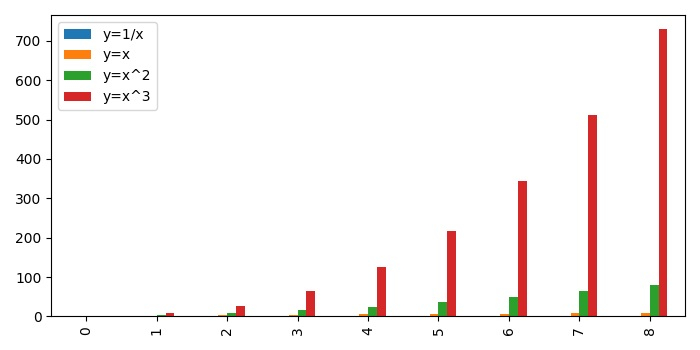# How to plot a bar graph in Matplotlib from a Pandas series?

To plot a bar graph from a Pandas series in matplotlib, we can take the following Steps −

• Make a dictionary of different keys, between the range 1 to 10.

• Make a dataframe using Pandas data frame.

• Create a bar plot using plot() method with kind="bar".

• To display the figure, use show() method.

## Example

import pandas as pd
from matplotlib import pyplot as plt
plt.rcParams["figure.figsize"] = [7.00, 3.50]
plt.rcParams["figure.autolayout"] = True
d = {'y=1/x': [1 / i for i in range(1, 10)],
'y=x': [i for i in range(1, 10)],
'y=x^2': [i * i for i in range(1, 10)],
'y=x^3': [i * i * i for i in range(1, 10)]}
df = pd.DataFrame(d)
df.plot(kind='bar')
plt.show()

## Output